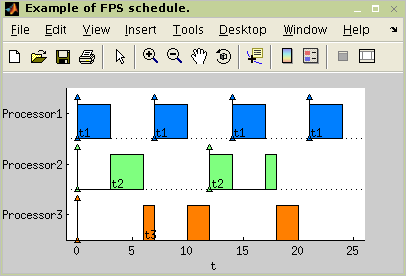"; } ?> Real-Time Scheduling

# TORSCHE Scheduling Toolbox for Matlab

## TORSCHE – Real-Time Scheduling

This section desribes how to use TORSCHE for analysis of real-time systems. The area of real-time scheduling is quite broad and currently only the basics are supported. We are working on addition of more advanced methods to the toolbox.

The real-time system we consider a set of periodic tasks (see Section 5, “Periodic Tasks”). The sections bellow describe various algorithms that work on sets of real-time tasks.

### 1. Fixed-Priority Scheduling

Algorithms in this section assume that the tasks have assigned fixed priority (property `Weight`). The higher number, the higher priority.

#### 1.1. Response-Time Analysis

The resptime function implements an algorithm that calculates response times for periodic tasks in a set. It is assumed, that these tasks are scheduled by a preemtive, fixed priority scheduler on one processor. Currently, this algorithm doesn't support any kind of synchronization between tasks. The syntax of the command is:

`[resp, schedulable] = resptime(taskset)`

where `resp` is array of response-times. There is one element for each task in the taskset. The parameter `schedulable` is non-zero if the system is schedulable, assuming the deadlines are equal to periods.

```>> t1=ptask('t1',3,7);
>> ts=[t1 t2 t3];
>> setprio(ts, 'rm');
>> [r,s]=resptime(ts)
r =
3    14    78
s =
1```

Figure 8.1. Calculating the response time using resptime

#### 1.2. Fixed-Priority Scheduler

Fixed Priority Scheduling (fps) is an algorithm that schedules periodic tasks in taskset according to their fixed priorities (property `Weight` of task).

`r = fps(TS)`

FPS algorithm is demonstrated on the example shown in next example code. The resulting schedule is shown in the next figure.

```>> t1=ptask('t1',3,7);   t1.Weight = 3;